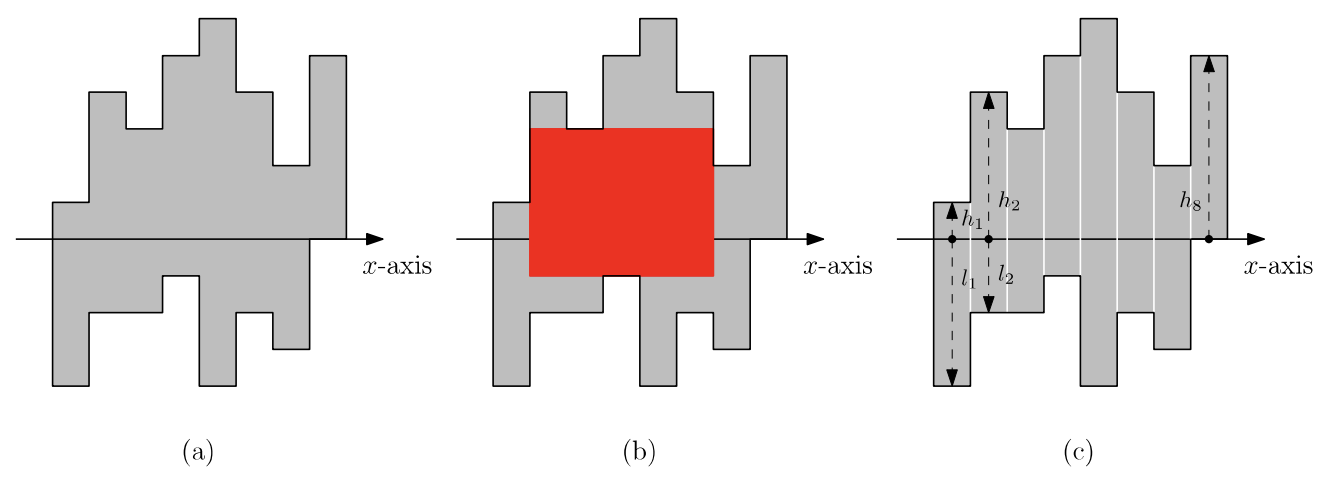시간 제한메모리 제한제출정답맞힌 사람정답 비율
2.5 초 (추가 시간 없음) 512 MB12611816.000%

## 문제

ISCO(ICPC Steel Company) is a company that buys steel sheets of a certain shape, cuts them into pieces, and sells them in the industry market. Every steel sheet that ISCO buys is a polygon without holes such that each side is either horizontal or vertical with respect to the x-axis. The length of each side is a positive integer. We call such a polygon a histogon. See Figure 1(a) for a histogon.Figure 1. (a) A steel sheet which is a histogon. (b) An axis-aligned rectangle (red) with maximum area contained in the histogon. (c) The histogon is subdivided along vertical lines into 8 rectangular slabs with width 1.

Since the market price of a piece becomes much higher if the piece is a rectangle with larger area, it is desirable to cut a steel sheet so as to get a rectangle of maximum area. Thus, the task is to find a rectangle contained in a steel sheet with largest area. We assume that the rectangle should be axis-aligned, that is, every side is horizontal or vertical with respect to the x-axis. Formally, this problem can be stated as follows. Given a histogon, find an axis-aligned rectangle with maximum area contained in the histogon. Figure 1(b) shows an axis-aligned rectangle with maximum area that is contained in the histogon in Figure 1(a).

A histogon with width n can be subdivided along vertical lines into n rectangular slabs with width 1. The histogon in Figure 1(a) can be subdivided along vertical lines into 8 rectangular slabs, as shown in Figure 1(c). These slabs are numbered from 1 to n in order along the x-axis such that the leftmost slab has number 1. To ease the description, we assume that the x-axis intersects every slab. The x-axis intersects every slab in Figure 1(c). Then, slab i can be represented by two values, hi and li, where hi denotes the vertical length of slab i lying above the x-axis and li denotes the vertical length of slab i lying below the x-axis.

Given a histogon with width n, write a program to output the maximum area among the axis-aligned rectangles contained in the histogon.

## 입력

Your program is to read from standard input. The input starts with a line containing one integer n (1 ≤ n ≤ 200,000), where n is the width of the input histogon. The slabs are numbered from 1 to n such that the leftmost slab has number 1. In the following n lines, the i-th line contains 2 nonnegative integers that represent hi and li (0 ≤ hi, li ≤ 1,000,000,000).

## 출력

Your program is to write to standard output. Print exactly one line. The line should contain the maximum area among rectilinear rectangles contained in the given histogon.

## 예제 입력 1

8
1 4
4 2
3 2
5 1
6 4
4 2
2 3
5 0


## 예제 출력 1

20


## 예제 입력 2

5
23 15
23 17
3 22
15 3
5 1


## 예제 출력 2

76